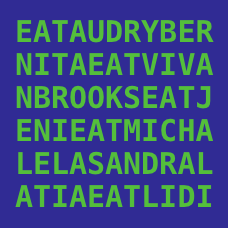Computer Science

# Join/Splitting/Substring

This text is an English translation of a poem by the famous French modernist Charles Baudelaire. If each vowel in the text $(a,e,i,o,u,y)$ has a frequency $F_i$, compute the last five digits of the product of all the frequencies of the vowels in the text. Specifically what is the value of the last five digits of $F_{'a'} \times F_{'e'} \times F_{'i'} \times F_{'o'} \times F_{'u'} \times F_{'y}'$?

NOTES:

• For the purposes of this problem, we'll count y as a vowel
• Don't forget to ignore case so both y and Y are vowels.

Write a program that finds the number of substrings of an integer that are divisible by $n$, where $n$ is an integer. For example, "8395", and "1376" are two substrings of "83951376".

How many substrings of 6781234967 are divisible by 3?

Substitution ciphers are ciphers in which each alphabetical character is mapped with another character in a one-to-one fashion. For example, the following string

the quick brown fox jumps over the lazy dog

when encrypted becomes

'fzq kpgwj vxbsa rbh lpnic bdqx fzq uomy ebt'

Based on the substitution above, your task is to decipher the text below. Once the text is deciphered, the answer shall be obvious.

'qafqx rbxfy fsb oc fzq oacsqx'

×